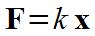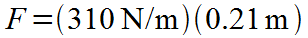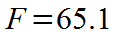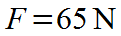### Calculate the amount of force necessary to stretch a linear spring a certain displacement given the spring constant.

#### Example question:

A linear spring has a constant of 310 N/m. How much force is necessary to stretch it 0.21 m?

#### Given:

spring constant: k = 310 N/m

displacement: x = 0.21 mStarting formulaPlug in numbers with unitsMultiply: (310)(0.21) = 65.1Final result with significant figures and unit